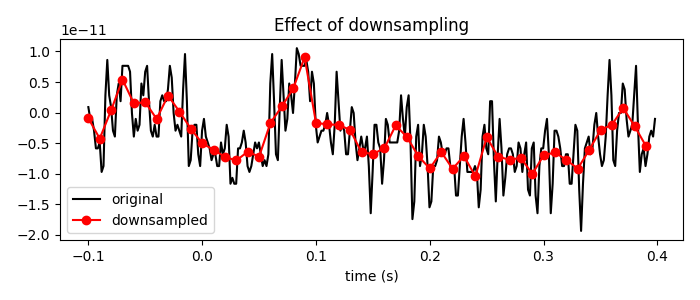# Resampling data¶

When performing experiments where timing is critical, a signal with a high sampling rate is desired. However, having a signal with a much higher sampling rate than is necessary needlessly consumes memory and slows down computations operating on the data.

This example downsamples from 600 Hz to 100 Hz. This achieves a 6-fold reduction in data size, at the cost of an equal loss of temporal resolution.

# Authors: Marijn van Vliet <w.m.vanvliet@gmail.com>
#

from matplotlib import pyplot as plt

import mne
from mne.datasets import sample


Setting up data paths and loading raw data (skip some data for speed)

data_path = sample.data_path()
raw_fname = data_path + '/MEG/sample/sample_audvis_raw.fif'


Out:

Opening raw data file /home/circleci/mne_data/MNE-sample-data/MEG/sample/sample_audvis_raw.fif...
Read a total of 3 projection items:
PCA-v1 (1 x 102)  idle
PCA-v2 (1 x 102)  idle
PCA-v3 (1 x 102)  idle
Range : 25800 ... 192599 =     42.956 ...   320.670 secs
Reading 0 ... 72074  =      0.000 ...   120.000 secs...


Since downsampling reduces the timing precision of events, we recommend first extracting epochs and downsampling the Epochs object:

events = mne.find_events(raw)
epochs = mne.Epochs(raw, events, event_id=2, tmin=-0.1, tmax=0.8, preload=True)

# Downsample to 100 Hz
print('Original sampling rate:', epochs.info['sfreq'], 'Hz')
print('New sampling rate:', epochs_resampled.info['sfreq'], 'Hz')

# Plot a piece of data to see the effects of downsampling
plt.figure(figsize=(7, 3))

n_samples_to_plot = int(0.5 * epochs.info['sfreq'])  # plot 0.5 seconds of data
plt.plot(epochs.times[:n_samples_to_plot],
epochs.get_data()[0, 0, :n_samples_to_plot], color='black')

n_samples_to_plot = int(0.5 * epochs_resampled.info['sfreq'])
plt.plot(epochs_resampled.times[:n_samples_to_plot],
epochs_resampled.get_data()[0, 0, :n_samples_to_plot],
'-o', color='red')

plt.xlabel('time (s)')
plt.legend(['original', 'downsampled'], loc='best')
plt.title('Effect of downsampling')
mne.viz.tight_layout()Out:

144 events found
Event IDs: [ 1  2  3  4  5 32]
33 matching events found
Applying baseline correction (mode: mean)
Created an SSP operator (subspace dimension = 3)
3 projection items activated
Original sampling rate: 600.614990234375 Hz
New sampling rate: 100.0 Hz


When resampling epochs is unwanted or impossible, for example when the data doesn’t fit into memory or your analysis pipeline doesn’t involve epochs at all, the alternative approach is to resample the continuous data. This can only be done on loaded or pre-loaded data.

# Resample to 300 Hz


Out:

144 events found
Event IDs: [ 1  2  3  4  5 32]
144 events found
Event IDs: [ 1  2  3  4  5 32]


Because resampling also affects the stim channels, some trigger onsets might be lost in this case. While MNE attempts to downsample the stim channels in an intelligent manner to avoid this, the recommended approach is to find events on the original data before downsampling.

print('Number of events before resampling:', len(mne.find_events(raw)))

# Resample to 100 Hz (suppress the warning that would be emitted)
print('Number of events after resampling:',
len(mne.find_events(raw_resampled_100)))

# To avoid losing events, jointly resample the data and event matrix
events = mne.find_events(raw)
raw_resampled, events_resampled = raw.copy().resample(
print('Number of events after resampling:', len(events_resampled))


Out:

144 events found
Event IDs: [ 1  2  3  4  5 32]
Number of events before resampling: 144
143 events found
Event IDs: [ 1  2  3  4  5 32]
Number of events after resampling: 143
144 events found
Event IDs: [ 1  2  3  4  5 32]
Number of events after resampling: 144


Total running time of the script: ( 0 minutes 8.565 seconds)

Estimated memory usage: 545 MB

Gallery generated by Sphinx-Gallery